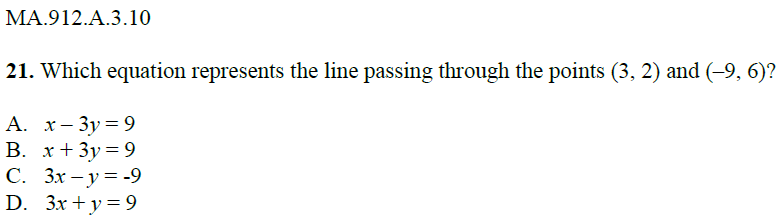# Question 21: Finding an Equation

Algebra Level 2MA.912.A.3.10 - Write an equation of a line given any of the following information: two points on the line, its slope and one point on the line, or its graph. Also, find an equation of a new line parallel to a given line, or perpendicular to a given line, through a given point on the new line.

Algebra 1 EOC Study Guide. How many can you solve correctly?

×

Problem Loading...

Note Loading...

Set Loading...